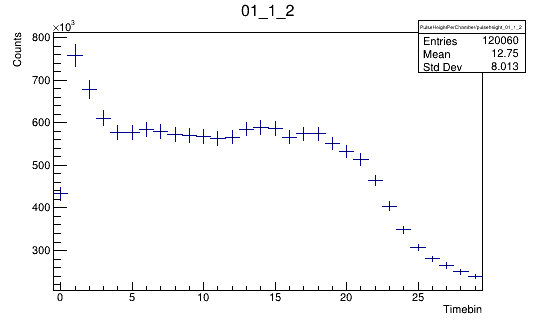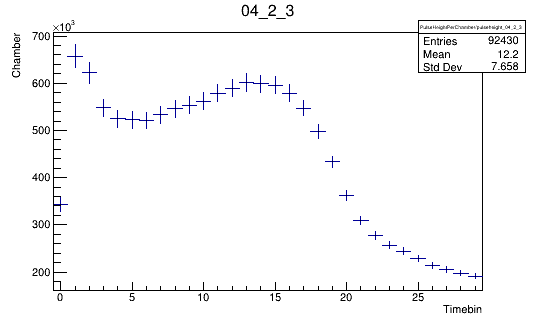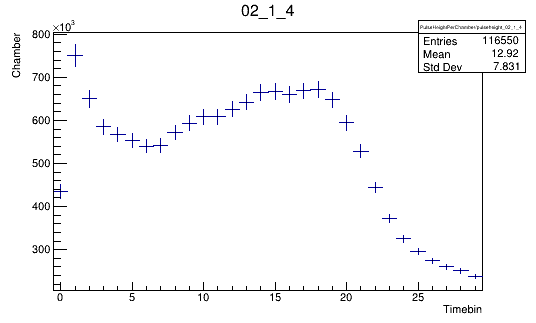# Fitting is not working on the triangular peak plot

Dear Experts,
I tried to fit this histogram with double exponential + constant function. We also tried several other functions. But I am not able to do a combine fit of this histogram. I have also attached the code.
Can you please suggest me how can I fit this histogram?

fit.C (1.1 KB)ROOT Version: Not Provided
Platform: Not Provided
Compiler: Not Provided

what you have looks definitely like a Fermi function/sigmoid/cumulative Gaussian (all equivalent anyway), plus maybe a single exponential at the beginning (or even another sigmoid on top).

Here is how you could define a sigmoid plus exponential function:

``````// testFunc.C

double myfunc(double *x, double *p) {

double u = p; // uniform plateau hight
double c = p; // coefficient for exponential
double tau = p; // exponential parameter
double mu = p; // Gaussian position
double sigma = p; // Gaussian width

using ROOT::Math::gaussian_cdf;
return u * ((1-gaussian_cdf(x, sigma, mu)) + c * std::exp(-tau * x));

}

void func() {

auto *f1 = new TF1("f1", myfunc, 0, 30.0, 5);
f1->SetTitle("Exponential plus cumulative Gaussian");
f1->SetParameters(580000.0, 0.5, 1.0, 22.0, 3.0);
f1->Draw();
}
``````

Hope this helps and gives you some ideas!
Jonas

1 Like

Dear Jonas,

I have tried this function and it is coming like this. I am not able to fit all the points.
What I want from this fitting is to extract some parameters such as slope of the constant region from 8 to 20.
I can somehow tweak the parameters so that fit looks good but the problem is that I have to do this for more than 500 histograms. Thus, I am looking for a general functions that will able to fit all the histograms which do not differ much. I am attaching some histos for reference.
Thanks